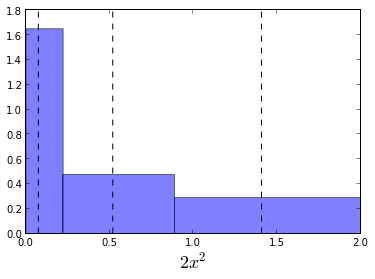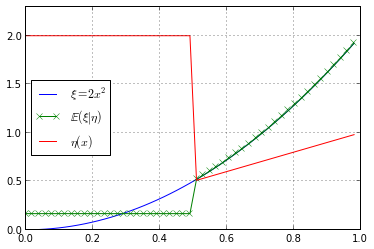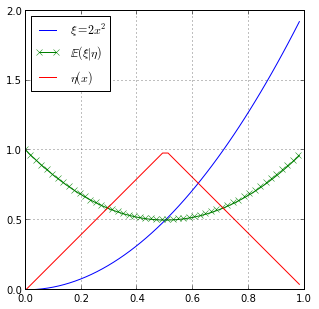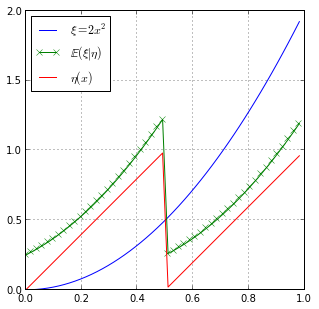## Introduction

Brzezniak (2000) is a great book because it approaches conditional expectation through a sequence of exercises, which is what we are trying to do here. The main difference is that Brzezniak takes a more abstract measure-theoretic approach to the same problems. Note that you do need to grasp the measure-theoretic to move into more advanced areas in stochastic processes, but for what we have covered so far, working the same problems in his text using our methods is illuminating. It always helps to have more than one way to solve any problem. I urge you to get a copy of his book or at least look at some pages on Google Books. I have numbered the examples corresponding to the book.

## Examples

This is Example 2.1 from Brzezniak:

Three coins, 10p, 20p and 50p are tossed. The values of those coins that land heads up are added to work out the total amount. What is the expected total amount given that two coins have landed heads up?

In this case we have we want to compute $\mathbb{E}(\xi|\eta)$ where

$$\xi = 10 X_{10} + 20 X_{20} +50 X_{50}$$

where $X_i \in { 0,1}$. This represents the sum total value of the heads-up coins. The $\eta$ represents the fact that only two of the three coins are heads-up. Note

$$\eta = X_{10} X_{20} (1-X_{50})+ (1-X_{10}) X_{20} X_{50}+ X_{10} (1-X_{20}) X_{50}$$

is a function that is only non-zero when two of the three coins is heads. Each triple term catches each of these three possibilities. For example, the first term is when the 10p and 20p are heads up and the 50p is heads down.

To compute the conditional expectation, we want to find a function $h$ of $\eta$ that minimizes the MSE

$$\sum_{X\in{0,1}^3} \frac{1}{8} (\xi - h( \eta ))^2$$

where the sum is taken over all possible triples of outcomes for ${X_{10} , X_{20} ,X_{50}}$ and the $\frac{1}{8} = \frac{1}{2^3}$ since each coin has a $\frac{1}{2}$ chance of coming up heads.

Now, the question boils down to what function $h(\eta)$ should we try? Note that $\eta \in {0,1}$ so $h$ takes on only two values. Thus, we only have to try $h(\eta)=\alpha \eta$ and find $\alpha$. Writing this out gives,

$$\sum_{X\in{0,1}^3} \frac{1}{8} (\xi - \alpha( \eta ))^2$$

which boils down to solving for $\alpha$,

$$\langle \xi , \eta \rangle = \alpha \langle \eta,\eta \rangle$$

where

$$\langle \xi , \eta \rangle =\sum_{X\in{0,1}^3} \frac{1}{8} (\xi \eta )$$

This is tedious and a perfect job for sympy.

In :
import sympy as S

eta = S.Symbol('eta')
xi = S.Symbol('xi')
X10 = S.Symbol('X10')
X20 = S.Symbol('X20')
X50 = S.Symbol('X50')

eta = X10 * X20 *(1-X50 )+ X10 * (1-X20) *(X50 )+ (1-X10) * X20 *(X50 )
xi = 10*X10 +20* X20+ 50*X50

num=S.summation(xi*eta,(X10,0,1),(X20,0,1),(X50,0,1))
den=S.summation(eta*eta,(X10,0,1),(X20,0,1),(X50,0,1))
alpha=num/den
print alpha

160/3


This means that

$$\mathbb{E}(\xi|\eta) = \frac{160}{3} \eta$$

which we can check with a quick simulation

In :
import numpy as np
from numpy import array

x=np.random.randint(0,2,(3,5000))
print (160/3.,np.dot(x[:,x.sum(axis=0)==2].T,array([10,20,50])).mean())

(53.333333333333336, 53.177920685959272)


## Example

This is example 2.2:

Three coins, 10p, 20p and 50p are tossed as before. What is the conditional expectation of the total amount shown by the three coins given the total amount shown by the 10p and 20p coins only?

For this problem,

$$\eta = 30 X_{10} X_{20} + 20 (1-X_{10}) X_{20} + 10 X_{10} (1-X_{20})$$

which takes on three values (10,20,30) and only considers the 10p and 20p coins. Here, we'll look for affine functions, $h(\eta) = a \eta + b$.

In :
from sympy.abc import a,b

eta = X10 * X20 * 30 + X10 * (1-X20) *(10 )+ (1-X10) * X20 *(20 )
h = a*eta + b

J=S.summation((xi - h)**2 * S.Rational(1,8),(X10,0,1),(X20,0,1),(X50,0,1))

sol=S.solve( [S.diff(J,a), S.diff(J,b)],(a,b) )
print sol

{b: 25, a: 1}


This means that

$$\mathbb{E}(\xi|\eta) = 25+ \eta$$

since $\eta$ takes on only four values, ${0,10,20,30}$, we can write this out as

$$\mathbb{E}(\xi|\eta=0) = 25$$ $$\mathbb{E}(\xi|\eta=10) = 35$$ $$\mathbb{E}(\xi|\eta=20) = 45$$ $$\mathbb{E}(\xi|\eta=30) = 55$$

The following is a quick simulation to demonstrate this.

In :
x=np.random.randint(0,2,(3,5000))  # random samples for 3 coins tossed
eta=np.dot(x[:2,:].T,array([10,20])) # sum of 10p and 20p

print np.dot(x[:,eta==0].T,array([10,20,50])).mean() # E(xi|eta=0)
print np.dot(x[:,eta==10].T,array([10,20,50])).mean()# E(xi|eta=10)
print np.dot(x[:,eta==20].T,array([10,20,50])).mean()# E(xi|eta=20)
print np.dot(x[:,eta==30].T,array([10,20,50])).mean()# E(xi|eta=30)

26.4120922832
34.5614035088
45.8196721311
55.3743104807


## Example

This is Example 2.3Note that "Lebesgue measure" on $[0,1]$ just means uniformly distributed on that interval. Also, note the the Piecewise object in sympy is not complete at this point in its development, so we'll have to work around that in the following.

In :
x=S.Symbol('x')
c=S.Symbol('c')

xi = 2*x**2

eta=S.Piecewise( (1, 0 < x< S.Rational(1,3)),
(2, S.Rational(1,3) < x< S.Rational(2,3)),
(0, S.Rational(2,3) < x < 1))

h = a + b*eta + c*eta**2
J=S.integrate((xi - h)**2 ,(x,0,1))

sol=S.solve( [S.diff(J,a),
S.diff(J,b),
S.diff(J,c),
],
(a,b,c) )
print sol

print S.piecewise_fold(h.subs(sol))

{c: 8/9, b: -20/9, a: 38/27}
Piecewise((2/27, x < 1/3), (14/27, x < 2/3), (38/27, x < 1))


Thus, collecting this result gives:

$$\mathbb{E}(\xi|\eta) = \frac{38}{27} - \frac{20}{9}\eta + \frac{8}{9} \eta^2$$

which can be re-written as a piecewise function as

$$\mathbb{E}(\xi|\eta) =\begin{cases} \frac{2}{27} & \text{for}\: 0 < x < \frac{1}{3} \\ \frac{14}{27} & \text{for}\: \frac{1}{3} < x < \frac{2}{3} \\ \frac{38}{27} & \text{for}\: \frac{2}{3}<x < 1 \end{cases}$$

The following is a quick simulation to demonstrate this.

In :
x = np.random.rand(1000)
f,ax= subplots()
ax.hist(2*x**2,bins=array([0,1/3.,2/3.,1])**2*2,normed=True,alpha=.5)
ax.vlines([2/27.,14/27.,38/27.],0,ax.get_ylim(),linestyles='--')
ax.set_xlabel(r'$2 x^2$',fontsize=18);This plot shows the intervals that correspond to the respective domains of $\eta$ with the vertical dotted lines showing the $\mathbb{E}(\xi|\eta)$ for that piece.

## Example

This is Example 2.4In :
x,a=S.symbols('x,a')

xi = 2*x**2

half = S.Rational(1,2)

eta_0=S.Piecewise( (2,    0 <= x < half),
(0, half <= x <= 1),
)

eta_1=S.Piecewise( (0,         x < half),
(x, half <= x <= 1),
)

v=S.var('b:3') # coefficients for quadratic function of eta

h = a*eta_0 + (eta_1**np.arange(len(v))*v).sum()

J=S.integrate((xi - h)**2 ,(x,0,1))
sol=S.solve([J.diff(i) for i in v+(a,)],v+(a,))
hsol = h.subs(sol)

f=S.lambdify(x,hsol,'numpy')
print S.piecewise_fold(h.subs(sol))

t = np.linspace(0,1,51,endpoint=False)

fig,ax = subplots()

ax.plot(t, 2*t**2,label=r'$\xi=2 x^2$')
ax.plot(t,[f(i) for i in t],'-x',label=r'$\mathbb{E}(\xi|\eta)$')
ax.plot(t,map(S.lambdify(x,eta_0+eta_1),t),label=r'$\eta(x)$')
ax.set_ylim(ymax = 2.3)
ax.grid()
ax.legend(loc=0);
#ax.plot(t,map(S.lambdify(x,eta),t))

Piecewise((1/6, x < 1/2), (2*x**2, x <= 1))

Out:
<matplotlib.legend.Legend at 0x4b2b910>The figure shows the $\mathbb{E}(\xi|\eta)$ against $\xi$ and $\eta$. Note that $\xi= \mathbb{E}(\xi|\eta)= 2 x^2$ when $x\in[0,\frac{1}{2}]$ . Assembling the solution gives,

$$\mathbb{E}(\xi|\eta) =\begin{cases} \frac{1}{6} & \text{for}\: 0 \le x < \frac{1}{2} \ 2 x^2 & \text{for}\: \frac{1}{2} < x \le 1 \end{cases}$$

This example warrants more a more detailed explanation since $\eta$ is more complicated. The first question is why did we choose $h(\eta)$ as a quadratic function? Since $\xi$ is a squared function of $x$ and since $x$ is part of $\eta$, we chose a quadratic function so that $h(\eta)$ would contain a $x^2$ in the domain where $\eta=x$. The motivation is that we are asking for a function $h(x)$ that most closely approximates $2x^2$. Well, obviously, the exact function is $h(x)=2 x^2$! Thus, we want $h(x)=2 x^2$ over the domain where $\eta=x$, which is $x\in[\frac{1}{2},1]$ and that is exactly what we have.

We could have used our inner product by considering two separate functions,

$\eta_1 (x) = 2$

where $x\in [0,\frac{1}{2}]$ and

$$\eta_2 (x) = x$$

where $x\in [\frac{1}{2},1]$. Thus, at the point of projection, we have

$$\mathbb{E}((2 x^2 - 2 c) \cdot 2) = 0$$

$$\int_0^{\frac{1}{2}} 2 x^2 \cdot 2 dx = \int_0^{\frac{1}{2}} c 2 \cdot 2 dx$$

and a solution for $c$,

$$c = \frac{1}{12}$$

Assembling the solution for $x\in[0,\frac{1}{2}]$ gives

$$\mathbb{E}(\xi|\eta) = \frac{2}{12}$$

We can do the same thing for the other piece, $\eta_2$,

$$\mathbb{E}((2 x^2 - c x^2) \cdot x) = 0$$

which, by inspection, gives $c=2$. Thus, for $x\in[\frac{1}{2},1]$ , we have

$$\mathbb{E}(\xi|\eta)= 2 x^2$$

which is what we had before.

## Example

This is Exercise 2.6In :
x,a=S.symbols('x,a')

xi = 2*x**2
eta = 1 - abs(2*x-1)

half = S.Rational(1,2)

eta=S.Piecewise(   (1+(2*x-1),    0 <= x < half),
(1-(2*x-1), half <= x < 1),
)

v=S.var('b:3') # assume h is quadratic in eta

h = (eta**np.arange(len(v))*v).sum()

J=S.integrate((xi - h)**2 ,(x,0,1))
sol=S.solve([J.diff(i) for i in v],v)
hsol = h.subs(sol)

print S.piecewise_fold(h.subs(sol))

t = np.linspace(0,1,51,endpoint=False)

fig,ax = subplots()
fig.set_size_inches(5,5)

ax.plot(t, 2*t**2,label=r'$\xi=2 x^2$')
ax.plot(t,[hsol.subs(x,i) for i in t],'-x',label=r'$\mathbb{E}(\xi|\eta)$')
ax.plot(t,map(S.lambdify(x,eta),t),label=r'$\eta(x)$')
ax.legend(loc=0)
ax.grid()

Piecewise((2*x**2 - 2*x + 1, x < 1/2), (2*x + (-2*x + 2)**2/2 - 1, x < 1))The figure shows that the $\mathbb{E}(\xi|\eta)$ is continuous over the entire domain. The code above solves for the conditional expectation using optimization assuming that $h$ is a quadratic function of $\eta$, but we can also do it by using the inner product. Thus,

$$\mathbb{E}\left((2 x^2 - h(\eta_1(x)) )\eta_1(x)\right)= \int_0^{\frac{1}{2}} (2 x^2 - h(\eta_1(x)) )\eta_1(x) dx = 0$$

where $\eta_1 = 2x$ for $x\in [0,\frac{1}{2}]$. We can re-write this in terms of $\eta_1$ as

$$\int_0^1 \left(\frac{\eta_1^2}{2}-h(\eta_1)\right)\eta_1 d\eta_1$$

and the solution jumps right out as $h(\eta_1)=\frac{\eta_1^2}{2}$. Note that $\eta_1\in[0,1]$. Doing the same thing for the other piece,

$$\eta_2 = 2 - 2 x, \hspace{1em} \forall x\in[\frac{1}{2},1]$$

gives,

$$\int_0^1 \left(\frac{(2-\eta_2)^2}{2}-h(\eta_2)\right)\eta_2 d\eta_2$$

and again, the optimal $h(\eta_2)$ jumps right out as

$$h(\eta_2) = \frac{(2-\eta_2)^2}{2} , \hspace{1em} \forall \eta_2\in[0,1]$$

and since $\eta_2$ and $\eta_2$ represent the same variable over the same domain we can just add these up to get the full solution:

$$h(\eta) = \frac{1}{2} \left( 2 - 2 \eta + \eta^2\right)$$

and then back-substituting each piece for $x$ produces the same solution as sympy.

## Example

This is Exercise 2.14In :
x,a=S.symbols('x,a')
half = S.Rational(1,2)

xi = 2*x**2
eta=S.Piecewise(   (2*x,    0 <= x < half),
((2*x-1), half <= x ),
)

v=S.var('b:3')

h = (eta**np.arange(len(v))*v).sum()

J=S.integrate((xi - h)**2 ,(x,0,1))
sol=S.solve([J.diff(i) for i in v],v)
hsol = h.subs(sol)

print S.piecewise_fold(h.subs(sol))

t = np.linspace(0,1,51,endpoint=False)

fig,ax = subplots()
fig.set_size_inches(5,5)

ax.plot(t, 2*t**2,label=r'$\xi=2 x^2$')
ax.plot(t,[hsol.subs(x,i) for i in t],'-x',label=r'$\mathbb{E}(\xi|\eta)$')
ax.plot(t,map(S.lambdify(x,eta),t),label=r'$\eta(x)$')
ax.legend(loc=0)
ax.grid()

Piecewise((2*x**2 + x + 1/4, x < 1/2), (x + (2*x - 1)**2/2 - 1/4, 1/2 <= x))As before, using the inner product for this problem, gives:

$$\int_0^1 \left(\frac{\eta_1^2}{2}-h(\eta_1)\right)\eta_1 d\eta_1=0$$

and the solution jumps right out as $$h(\eta_1)=\frac{\eta_1^2}{2} , \hspace{1em} \forall \eta_1\in[0,1$$

where $\eta_1(x)=2x$. Doing the same thing for $\eta_2=2x-1$ gives,

$$\int_0^1 \left(\frac{(1+\eta_2)^2}{2}-h(\eta_2)\right)\eta_1 d\eta_2=0$$

with

$$h(\eta_2)=\frac{(1+\eta_2)^2}{2} , \hspace{1em} \forall \eta_2\in[0,1]$$

and then adding these up as before gives the full solution:

$$h(\eta)= \frac{1}{2} +\eta + \eta^2$$

Back-substituting each piece for $x$ produces the same solution as sympy.

In :
xs = np.random.rand(100)
print np.mean([(2*i**2-hsol.subs(x,i))**2 for i in xs])
print S.integrate((2*x**2-hsol)**2,(x,0,1)).evalf()

0.266422848567040
0.270833333333333


## Summary

We worked out some of the great examples in Brzezniak's book using our methods as a way to show multiple ways to solve the same problem. In particular, comparing Brzezniak's more measure-theoretic methods to our less abstract techniques is a great way to get a handle on those concepts which you will need for more advanced study in stochastic process.

As usual, the corresponding IPython Notebook notebook for this post is available for download here